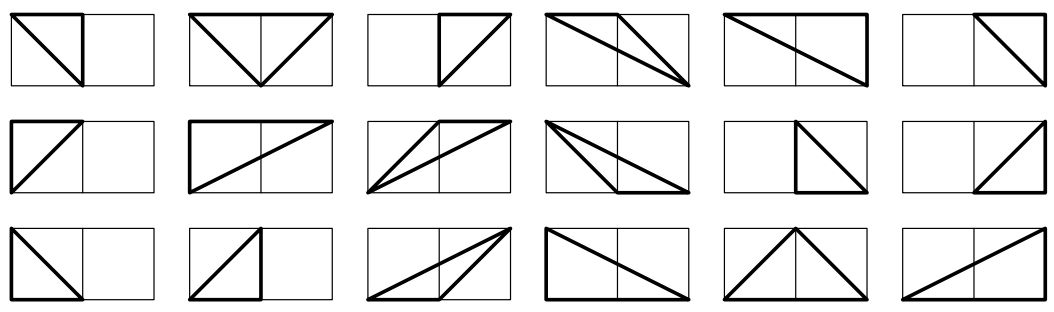시간 제한 메모리 제한 제출 정답 맞은 사람 정답 비율
2 초 512 MB 0 0 0 0.000%

## 문제

Triangles are polygons with three sides and strictly positive area. Lattice triangles are the triangles all whose vertices have integer coordinates. In this problem you have to find the number of lattice triangles in an M × N grid. For example in a 1 × 2 grid there are 18 different lattice triangles as shown in the picture below:Figure 2: Lattice Triangles in a 1 × 2 Grid

## 입력

The input file contains at most 21 sets of inputs.

Each set of input consists of a line that contains two integers M and N (0 < M, N ≤ 1000). These two integers denote that you have to count triangles in an M × N grid.

The input is terminated by a case where the value of M and N are zero. This case should not be processed.

## 출력

For each set of input produce one line of output. This output contains the case number of output followed by the number of lattice triangles in the grid. You can assume that the number of triangles will fit in a 64-bit signed integer.

## 예제 입력 1

1 1
1 2
0 0


## 예제 출력 1

Case 1: 4
Case 2: 18